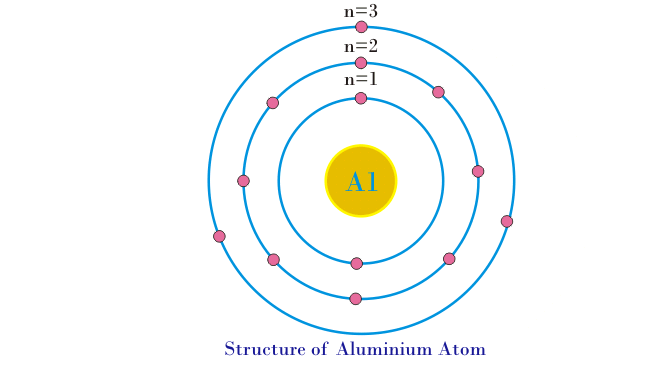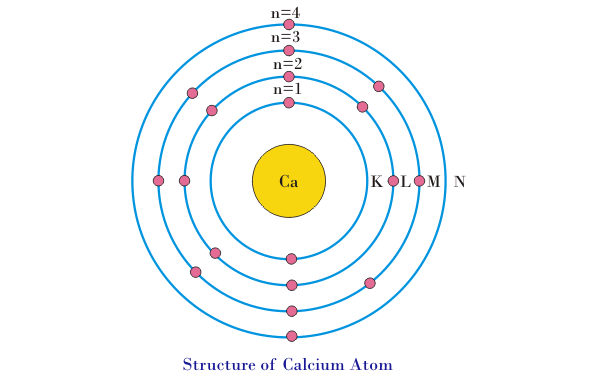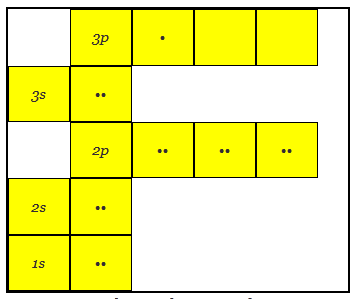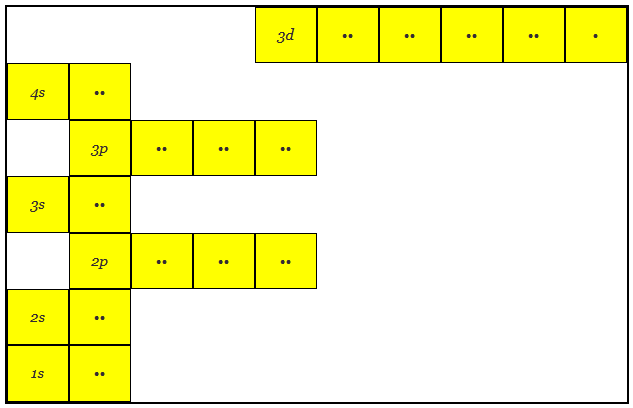# What is an Atom?

An atom is defined as the smallest particle of a substance that can exist by itself or be combined with other atoms to form a molecule.

In the year 1808, famous English chemist, physicist, and meteorologist John Dalton published his theory of the atom. At that time, many unexplained chemical phenomena got quickly unlocked by Dalton’s theory. Hence, the theory became a theoretical foundation of chemistry. The postulates of Dalton’s atomic theory were as follows.

• All matter is made up of small indivisible and indestructible particles called atoms.
• All atoms of the same element have identical properties but differ from atoms of other elements.
• Atoms of different elements combine together to form a compound.
• A chemical reaction is nothing but a rearrangement of these atoms.
• Atoms cannot be created or destroyed by any means.

Dalton’s theory had certain drawbacks like; today we know that atoms can be destroyed. Also, some atoms of the same elements vary in their mass(isotopes). The theory also fails to explain the existence of allotropes.

But in mordern era concept of atom is based on the combining the merits of Rutherford’s atomic model and Bohr’s atomic model. All substances are made up of atoms. All atoms consist of,

1. Nucleus
2. Electrons

## Nucleus of Atom

Nucleus is located at the center of atom. The diameter of nucleus is about 1/10000 of diameter of whole atom. Almost whole mass of atom is concentrated in its nucleus. Nucleus itself consists of two kinds of particles,

1. Proton
2. Neutron

### Proton

Protons are positively charged particles. Charge on each proton is 1.6 × 10-19 Coulomb. The number of protons in nucleus of an atom represents the atomic number of atom.

### Neutron

Neutrons do not have any electrical charge. Means, neutrons are electrically neutral particles. The mass of each neutron is equal to mass of the proton.
The nucleus is positively charged due to the presence of positively charged protons. In any material, the weight of the atom and radioactive properties are associated with the nucleus.

## Electrons

An electron is a negatively charged particle present in the atoms. Charge on each electron is – 1.6 × 10 – 19 Coulomb. These electrons surround the nucleus. Some facts about electrons in an atom are listed and explained below,

• If an atom is having the same number of protons and electrons, the atom is electrically neutral as the negative charge of electrons neutralizes the positive charge of protons.
• The electrons revolve around the nucleus in shells (also called orbits).
• A force of attraction is excreted on negatively charged electrons by positively charged nucleus. This force of attraction works as centripetal force required for electrons revolution around the nucleus.
• The electrons which are near to nucleus are tightly bound with the nucleus and it is more difficult to pull out (remove) these electrons from the atom than those which are far away from the nucleus.
• The structure of aluminum atoms is shown in figure below-• A definite amount of energy is required to remove the electron from its orbit. The energy required to remove the electron from the first orbit is much more as compared to the energy required to remove the electron from the outer orbit. This is due to the force of attraction excreted by nucleus on electrons in the first orbit is much more as compared to the force of attraction excreted on electrons of outer orbit. Similarly, the energy required to remove the electron from the second orbit will be less as compared to the first orbit and greater than the third orbit. Hence, we can say that the electrons in the orbit are associated with a definite amount of energy. Thus the orbits or shells are also referred as energy levels.
• The energy levels are denoted by the letters K, L, M, N, etc. Where, K is the nearest orbit to the nucleus and having lowest energy level. Conversely, the outermost orbit is having highest energy level.
• The maximum number of electrons in any energy level is given by, ‘2n2’, where, n is an integer and represents the “principal quantum number”. For different energy levels the value of ‘n’ and maximum number of electrons as given in table below
 Sl. No. Energy level or Orbit (shell) Principal quantum number ‘n’ Maximum Number of electrons (2n2) 1 K 1 2 × 12 = 2 2 L 2 2 × 22 = 8 3 M 3 2 × 32 = 18 4 N 4 2 × 42 = 32
• The above formula (2n2) used for determining the maximum number of electrons in any shell, has some limitations. The Number of electrons in outermost shell (highest energy level) cannot exceed beyond 8. For example let’s consider the atom of calcium, it is having 20 electrons orbiting around its nucleus. As per formula of above rule i.e. 2n2, the electrons distribution will be as 2 electrons in K level, 8 electrons in L level and there will be balance 10 electrons. But the electrons in outer most energy level cannot exceed 8. Hence, there will be 8 electrons in M level and balance 2 electrons will go to next energy level i.e. 2 electrons will go the N level. The electron configuration of Calcium atom is shown in figure below-• The electrons in the outermost energy level are called “valence electrons”. The maximum possible number of “valence electrons” is 8. If the number of electrons in outermost orbit is 8, the atom becomes stable. The material whose atoms naturally having 8 electrons in their outermost shell (orbit), does not react with other material. Inert gases such as Helium, Neon, Argon, krypton etc. are having completely filled outer most shells and hence do not react with other materials.
• The “valence electrons” of an atom are loosely bound with the nucleus and can be freed / removed by different method i.e. by heating, by applying electric voltage etc.
• The “valence electrons” form the bonds to hold the atoms of material together. Most of the properties of materials are determined by these bonds in materials.
• The each main shell (energy level) is subdivided into sub shells. These sub shell are called orbitals. These sub shell /orbitals are designated by s, p, d, f etc. with corresponding quantum number, l = 0, 1, 2, 3, 4,….(n-1) etc. The number of sub shells in any main shell is equal to principal quantum number ‘n’. The electron capacity of any main shell can be determined by adding the electron capacity of sub shells. The maximum electron capacity of sub shells is governed with the formula of 2(2l +1 ). The capacities of sub shells are given in table below-
 Sl. No. Subshell Quantum number(l) Electron capacity of subshell 2(2l + 1) 1 s 0 2(2 × 0 + 1) = 2 2 p 1 2(2 × 1 + 1) = 6 3 d 2 2(2 × 2 + 1) = 10 4 f 3 2(2 × 3 + 1) = 14
• First shell i.e. K shell will have principal quantum number 1 and one s orbital and hence the s orbital is denoted as 1s.
• The second shell i.e. L shell will have principal quantum number 2 and will have one s and one p orbital and they are denoted as 2s and 2p respectively.
• The third shell i.e. M shell will have principal quantum number 3 and will have one s, one p, and one d orbital and they are denoted as 3s, 3p and 3d respectively and so on.
• Here we have another thing to remember that s orbital has one suborbital and every sub-orbital can contain maximum two electrons. The p orbital has 3 suborbital and d orbital has 5 suborbital.
• The lower energy suborbital are first filled up and then next higher orbital is filled. There would not be any chance of filling up any higher orbital or suborbital before filling is completed in its lower orbital.

If we go through the examples below it will be clear to us.

### Atomic Structure of Aluminum having 13 Electrons### Atomic Structure of Copper having 29 ElectronsHere it can be noticed that 3d orbital is in higher energy level than 4s

### Atomic Structure of Silver having 47 ElectronsHere it can be noticed that 3d orbital is in higher energy level than 4s similarly 4d orbital is in higher energy level than 5s similarly.

### Modern Atomic Theory

The modern atomic theory is just little more evolved than the Dalton’s Theory. Modern atomic theory is also called as quantum theory. The concept of wave particle duality comes into picture here. It says that the electrons which are considered to be particles can sometimes behave like waves. So an atom has a nucleus which is surrounded by probability clouds. These clouds are the most probably locations of electrons. The size and shape of these clouds can be calculated by using the equations of the waves.

Want To Learn Faster? 🎓
Get electrical articles delivered to your inbox every week.
No credit card required—it’s 100% free.